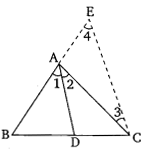" ">

# In the given figure, $D$ is a point on side $BC$ of $âˆ†ABC$, such that $\frac{BD}{CD}=\frac{AB}{AC}$. Prove that $AD$ is the bisector of $âˆ†BAC$."

Given:

$D$ is a point on side $BC$ of $∆ABC$, such that $\frac{BD}{CD}=\frac{AB}{AC}$.

To do:

We have to prove that $AD$ is the bisector of $∆BAC$.

Solution:

Produce $BA$ to $E$ such that $AE=EC$ and join $EC$.$\frac{\mathrm{BD}}{\mathrm{DC}}=\frac{\mathrm{AB}}{\mathrm{AC}}$

$\frac{\mathrm{BD}}{\mathrm{DC}}=\frac{\mathrm{AB}}{\mathrm{AE}}$             ($\mathrm{AC}=\mathrm{AE}$)

This implies,

$DA \| CE$        (By converse of Thales Theorem)

Therefore,

$\angle 2 =\angle 3$........(i)      (Alternate angles)

$\angle 1 =\angle 4$........(ii)      (Corresponding angles)

$\mathrm{AE}=\mathrm{AC}$

This implies,

$\angle 3=\angle 4$.........(iii)

From (i), (ii) and (iii), we get,

$\angle 1=\angle 2$

This implies,

$\mathrm{AD}$ is the bisector of $\angle \mathrm{BAC}$.

Hence proved.

Updated on: 10-Oct-2022

17 Views